\$B\$+\$s\$?\$s@_Dj\$N4pK\A`:nNc(B

\$B@_Dj\$r;2>H\$9\$k(B/\$B@_Dj\$r3NG'\$9\$k(B

 \$B:n@.F|(B 2000/Feb/22 \$B:G=*JQ99F|(B 2018/Nov/06 \$BJ8=q%5%\$%:(B 29KB

[ \$BL\

• \$B9)>l=P2Y>uBV\$N@_Dj(B
• \$BC

[ \$B\$+\$s\$?\$s@_Dj\$G@_Dj\$r3NG'\$9\$k(B ]

• \$B9)>l=P2Y>uBV\$N@_Dj\$r\$+\$s\$?\$s@_Dj\$G3NG'(B

1. WWW\$B%V%i%&%6\$G!"%k!<%?\$N!V\$+\$s\$?\$s@_Dj%Z!<%8!W\$r3+\$3\$&!*(B

\$B@_DjMQ%3%s%T%e!<%?\$r(BDHCP\$B\$K\$h\$k(BIP\$B%"%I%l%9<+F0 WWW\$B%V%i%&%6\$G!V(Bhttp://192.168.0.1/\$B!W\$K%"%/%;%9\$7\$^\$9!#(B

2. \$B%Q%9%o!<%I\$N@_Dj2hLL\$,I=<(\$5\$l\$^\$9!#(B

\$B#2%v=j\$N%Q%9%o!<%IF~NOMs\$KF1\$8%Q%9%o!<%I\$r@_Dj\$7\$F(B \$B%\%?%s\$rA*Br\$7\$^\$9!#(B

YAMAHA Remote Router RTA50i Rev.3.04.44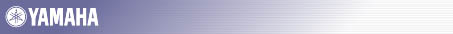RTA50i \$B\$+\$s\$?\$s@_Dj%Z!<%8\$X9T\$/A0\$K(B

\$B%"%/%;%9\$9\$k\$?\$a\$N%Q%9%o!<%I\$r@_Dj\$7\$^\$9!#H>3Q\$G(B8\$BJ8;z0JFb\$GF~NO\$7\$F\$/\$@\$5\$\$!#(B

 \$B%Q%9%o!<%I(B
\$B3NG'\$N\$?\$a!"F1\$8\$b\$N\$r\$b\$&0lEYF~NO\$7\$F\$/\$@\$5\$\$!#(B
 \$B%Q%9%o!<%I(B

 \$BCm0U(B\$B!'(B \$B\$3\$N%Q%9%o!<%I\$O!":#8e\$+\$s\$?\$s@_Dj%Z!<%8\$K%"%/%;%9\$9\$k:]\$KI,MW\$H\$J\$j\$^\$9\$N\$G!"K:\$l\$J\$\$\$h\$&\$KCm0U\$7\$F\$/\$@\$5\$\$!#%"%/%;%9;~\$K\$O!V%f!<%6L>!W\$H!V%Q%9%o!<%I!W\$,MW5a\$5\$l\$^\$9\$,!"!V%f!<%6L>!W\$O6uMs\$H\$7!"%Q%9%o!<%I\$N\$_\$rF~NO\$7\$F\$/\$@\$5\$\$!#(B

\$B

3. \$B@5\$7\$/@_Dj\$5\$l\$k\$H3NG'\$N2hLL\$,I=<(\$5\$l\$^\$9!#(B

\$B\$3\$3\$G(B\$B%\%?%s\$rA*Br\$9\$k\$H(B WWW\$B%V%i%&%6\$K\$h\$j!V\$+\$s\$?\$s@_Dj!W\$K%"%/%;%9\$9\$k%Q%9%o!<%I\$,5a\$a\$i\$l\$^(B \$B\$9!#(B

 \$B%Q%9%o!<%I\$N@_Dj(B \$B%Q%9%o!<%I\$r@_Dj\$7\$^\$7\$?!#(B \$B!V(B OK \$B!W%\%?%s\$r2!\$9\$H:FEY%Q%9%o!<%I\$NF~NO\$,5a\$a\$i\$l\$^\$9!#!V%f!<%6L>!W\$O6uMs\$N\$^\$^\$H\$7!"@_Dj\$7\$?%Q%9%o!<%I\$N\$_\$rF~NO\$7\$F\$/\$@\$5\$\$!#(B \$B%H%C%W%a%K%e!<\$X(B

4. WWW\$B%V%i%&%6\$N%Q%9%o!<%IF~NO2hLLNc(B

\$B%M%C%H%o!<%/%Q%9%o!<%I\$NF~NO(B
 \$B%f!<%6L>\$H%Q%9%o!<%I\$rF~NO\$7\$F\$/\$@\$5\$\$!#(B \$B%5%\$%H(B 192.168.0.1 \$BNN0h(B YAMAHA RT \$B%f!<%6L>(B(U) \$B%Q%9%o!<%I(B(P) \$B\$3\$N%Q%9%o!<%I\$rJ]B8\$9\$k(B

5. \$B!V\$+\$s\$?\$s@_Dj%Z!<%8\$X\$h\$&\$3\$=!W\$,I=<(\$5\$l\$^\$9!#(B

YAMAHA Remote Router RTA50i Rev.3.04.44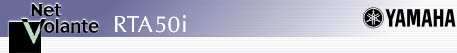RTA50i \$B\$+\$s\$?\$s@_Dj%Z!<%8\$X\$h\$&\$3\$=(B

\$B\$3\$N%Z!<%8\$G\$O(BRTA50i\$B\$N4pK\E*\$J@_Dj\$dA`:n\$,\$G\$-\$^\$9!#%k!<%?\$H\$7\$F\$*;H\$\$\$N>l9g\$O!"Cl9g\$G\$b!"7@Ls\$,Cl9g\$OC\$l\$N2hLL\$X?J\$_\$^\$9!#(B

 \$B%k!<%?\$H\$7\$F;HMQ\$9\$k>l9g\$NI,?\;v9`\$G\$9!#(BTA\$B\$H\$7\$F\$N\$_;H\$&>l9g\$OI,MW\$"\$j\$^\$;\$s!#%W%m%P%\$%@\$H\$N7@Ls\$K1~\$8\$FA*\$s\$G\$/\$@\$5\$\$!#(B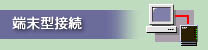\$B8D?M\$G%W%m%P%\$%@\$K2CF~\$7\$F\$\$\$k>l9g\$O\$[\$H\$s\$I\$,\$3\$N@\B3\$G\$9!#%@%\$%d%k%"%C%W\$9\$k\$?\$S\$KM?\$(\$i\$l\$k\$R\$H\$D\$N(BIP\$B%"%I%l%9\$r!"(BLAN\$BB&\$N5!4o\$G6&M-\$7\$^\$9!#(B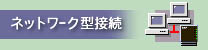\$B%W%m%P%\$%@\$+\$i8GDjE*\$KJ#?t\$N(BIP\$B%"%I%l%9\$rM?\$(\$i\$l\$F\$\$\$k>l9g\$N@\B3\$G\$9!#%k!<%?\$N(BIP\$B%"%I%l%9\$rJQ99\$7\$?8e\$O!"\$=\$l\$>\$l\$N(BPC\$B\$N(BIP\$B%"%I%l%9\$NJQ99\$bI,MW\$G\$9!#(B \$B%*%W%7%g%s;v9`\$G\$9!#(B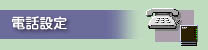\$B3F(BTEL\$B%]!<%H\$N%@%\$%d%k%\$%sHV9f\$d%5%V%"%I%l%9!"(Bi\$B!&%J%s%P!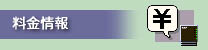\$B%G!<%?DL?.\$d3F(BTEL\$B%]!<%H\$NNA6b\$r3NG'\$7\$^\$9!#(B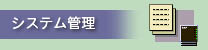\$B%a!<%kCe?.3NG'!?E>Aw5!G=\$d(BRVS-COM\$B!"%Q%9%o!<%I!";~9o\$J\$I\$r@_Dj\$7\$^\$9!#%a%s%F%J%s%9MQ\$NFbIt>pJs\$b3NG'\$G\$-\$^\$9!#(B

6. \$B9)>l=P2Y>uBV\$XLa\$7\$?D>8e\$N@_Dj\$r3NG'\$9\$k\$?\$a\$K!"(B \$B\$^\$:!"%7%9%F%`4IM}2hLL\$rI=<(\$7\$^\$9!#(B\$B%"%\$%3%s(B(\$B2hA|(B)\$B\$rA*Br\$7\$^\$9!#(B

YAMAHA Remote Router RTA50i Rev.3.04.44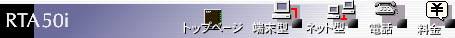\$B%7%9%F%`4IM}(B

 ISDN\$B2s@~\$r;HMQ\$7\$F%a!<%k%A%'%C%/\$r9T\$J\$&>l9g\$O2]6b\$K\$4Cm0U\$/\$@\$5\$\$!#(B
\$B%a!<%k%5!<%P\$NEPO?(B  4\$B\$D\$^\$GEPO?\$G\$-\$^\$9!#%a!<%k\$NCe?.\$r3NG'\$9\$k\$H!"K\BN\$N(BL1\$B%i%s%W\$,E@LG\$7\$^\$9!#(B

 LAN\$B@\B3HG(BRVS-COM(ISDN-DCP)\$B\$NCe?.@_Dj(B LAN\$B@\B3HG(BRVS-COM(ISDN-DCP)\$B\$NCe?.\$r(B \$B5v2D\$7\$J\$\$(B \$B5v2D\$9\$k(B RVS-COM\$B@_Dj(B

 \$B%k!<%?\$K%"%/%;%9\$9\$k;~\$N%Q%9%o!<%I(B \$BF1\$8\$b\$N\$r\$b\$&0lEY(B \$B%k!<%?\$N(BISDN\$BHV9f(B ISDN\$BHV9f(B \$B%5%V%"%I%l%9(B \$BCe?.\$r5v2D\$9\$k(Bi\$B!&%J%s%P!<\$N%]!<%HHV9f!!(B \$B%]!<%H(B1 \$B%]!<%H(B2 \$B%k!<%?\$K@_Dj\$9\$kF|;~(B \$BF|;~\$rJQ99\$7\$J\$\$(B \$B2<5-;XDjF|;~\$KJQ99\$9\$k(B \$BG/(B \$B7n(B \$BF|(B \$B;~(B \$BJ,(B \$BIC(B

\$B>e5-\$N9`L\\$r@_Dj\$7\$?8e!"EPO?%\%?%s\$G@_DjFbMF\$rEPO?\$7\$F\$/\$@\$5\$\$!#(B

7. \$B2hLL\$NI=<((B\$B2hLL\$G(B \$B%\%?%s\$rA*Br\$7\$^\$9!#(B
\$B2hLL\$G\$O!"(B \$B%3%^%s%IF~NO(B\$B\$d(B \$B@_Dj(B\$B\$H(B\$B%m%0(B\$B\$,;2>H\$G\$-\$^\$9!#(B\$B%7%9%F%`4IM}!'FbIt>pJs(B

 Config\$BI=<((B Syslog\$B\$X(B \$B@_DjFbMF\$rI=\$o\$9\$b\$N\$G\$9!#D>@\(B\$B%3%^%s%I\$rF~NO(B\$B\$7\$F@_Dj\$rJQ99\$9\$k\$3\$H\$b\$G\$-\$^\$9!#(B
```# RTA50i Rev.3.04.44 (Fri Jan 14 09:42:53 2000)
#  Memory 4Mbytes, 1LAN, 1BRI
dhcp service server
dhcp scope 1 192.168.0.2-192.168.0.191/24
analog supplementary-service pseudo call-waiting
```
 \$B%3%^%s%IF~NO(B Config\$B\$X(B \$B%V%i%&%6\$GF~NO2DG=\$J%3%^%s%I\$K\$O@)8B\$,\$"\$j\$^\$9!#(Bping \$B\$d(B traceroute \$B\$J\$I\$N

 Syslog\$BI=<((B Config\$B\$X(B \$B3FuBV\$d%Q%1%C%H\$N\$d\$j\$H\$j\$rI=\$o\$9\$b\$N\$G\$9!#(B\$B!V:G?7>pJs!W%\%?%s\$G99?7\$7\$^\$9!#%V%i%&%6\$N:FFI\$_9~\$_(B/\$B99?7%\%?%s\$G\$b99?7\$5\$l\$^\$9!#(B
```2000/02/22 15:21:01: Configure save in Nonvolatile memory
2000/02/22 15:21:03: RTA50i Rev.3.04.44 (Fri Jan 14 09:42:53 2000) starts
2000/02/22 15:28:41: Configure save in Nonvolatile memory
```

8. \$B2hLL\$NI=<(\$r=*\$k!#(B

\$B2hLL\$NI=<(\$r=*N;\$9\$k>l9g\$K\$O!"(B \$B\$rA*Br\$7\$^\$9!#(B

• \$B9)>l=P2Y>uBV\$N@_Dj\$rHf3S\$7\$F\$_\$h\$&!#(B

• \$B\$+\$s\$?\$s@_Dj\$G3NG'\$7\$?!V9)>l=P2Y>uBV\$N@_Dj!W(B

 ```# RTA50i Rev.3.04.44 (Fri Jan 14 09:42:53 2000) # MAC Address : 00:a0:de:00:00:fa # Memory 4Mbytes, 1LAN, 1BRI login password * administrator password * ip lan address 192.168.0.1/24 dhcp service server dhcp scope 1 192.168.0.2-192.168.0.191/24 analog supplementary-service pseudo call-waiting ```

• \$B%3%s%=!<%k(B(\$B%7%j%"%k\$d(Btelnet)\$B\$G3NG'\$7\$?!V9)>l=P2Y>uBV\$N@_Dj!W(B

\$B\$3\$N@_Dj\$O!"%3%s%=!<%k(B(\$B%7%j%"%k\$d(Btelnet)\$B\$G!"3NG'\$9\$k\$3\$H\$,\$G\$-\$^\$9\$,!"(B \$B\$+\$s\$?\$s@_Dj\$G3NG'\$7\$?FbMF(B(\$B2<@~ItJ,(B)\$B\$H\$O!"0[\$J\$C\$F\$\$\$k\$3\$H\$,\$o\$+\$j\$^\$9!#(B

 ```# RTA50i Rev.3.04.44 (Fri Jan 14 09:42:53 2000) # MAC Address : 00:a0:de:00:00:fa # Memory 4Mbytes, 1LAN, 1BRI ip lan address 192.168.0.1/24 dhcp service server dhcp scope 1 192.168.0.2-192.168.0.254/24 analog supplementary-service pseudo call-waiting ```

• \$B\$+\$s\$?\$s@_Dj%Z!<%8\$,3+\$1\$J\$\$!*(B

[ \$B4XO"\$9\$k(BRTA50i\$BMQ@_DjNc(B ]

[ \$B4XO">pJs(B ]

[ RTA50i (FAQ , \$B@_DjNc=8(B / \$B\$+\$s\$?\$s@_Dj\$N4pK\A`:n(B (files) ]